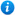* Watermarks do not appear on purchased resources

Preview resource: Click slides to enlarge

# Multiply Fractions

KS2 Maths Teaching Resources: Multiply Fractions

Year 5 maths programme of study - Number (fractions):

• multiply proper fractions and mixed numbers by whole numbers

In this maths teaching resource, pupils learn how to multiply proper fractions and mixed numbers by whole numbers.

'Multiply Fractions' is an animated PowerPoint presentation that contains:

• interactive activities
• a link to fractions word problems activities

This maths teaching resource is fully editable so that teachers have the freedom to adapt it to suit their individual teaching requirements.

'Multiply Fractions' can be previewed by clicking on the images opposite.

Our Price : £1.99 / 2 Credits